# How to go from device coordinates back to worldspace in OpenGL (with explanation)

back to index

So you’re writing a 3D engine or somesuch in OpenGL (modern or legacy), and you’re not a beginner so you know that the worldspace coordinates get transformed using your modelview/projection matrix (which we’ll treat as one for the purpose of this article) into clip space, which gets transformed by the perspective division into normalized device coordinates.

If you don’t, go read http://unspecified.wordpress.com/2012/06/21/calculating-the-gluperspective-matrix-and-other-opengl-matrix-maths/, it’s really good.

So you end up with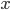,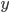and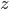(depth buffer) values; that is, device coordinates. But for some occasions, like shadow mapping or deferred lighting, it’s useful to do this transformation backwards - going from device coordinates back to worldspace. In this article, I’m going to walk you through the math of it.

Let’s start with what we have. We start out with our world space position,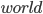. Note that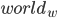is assumed to be 1 - for the rationale, see the article linked above.

We transform it to clip space by multiplying it with our projection/modelview matrix.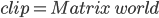Then move on to device coordinates by dividing with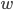.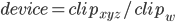So the problem we face is: given,,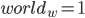,

and given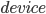as an input and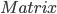as a constant, calculate.

Let’s walk through it. Invert the first step: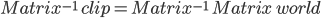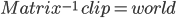Now let’s see what we can do with the second equation.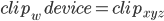Let’s use this syntax to indicate a 4-vector formed by combining a 3-vector and a fourth number: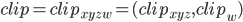substitute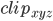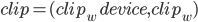insert into our earlier equation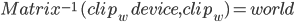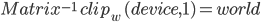And note that since matrices are linear transforms, we can pull that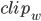in front of the matrix multiply: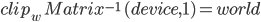So it seems we run into a wall.is lost, right? Don’t give up hope: we haven’t used the third of our initial givens yet.So let’s look at just thecomponent of that last equation there: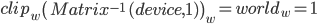Divide: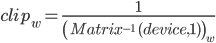And insert into the equation that previously gave us trouble: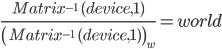Or in other words: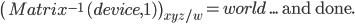You’ve probably seen that equation in shader code before. Well, now you know why.